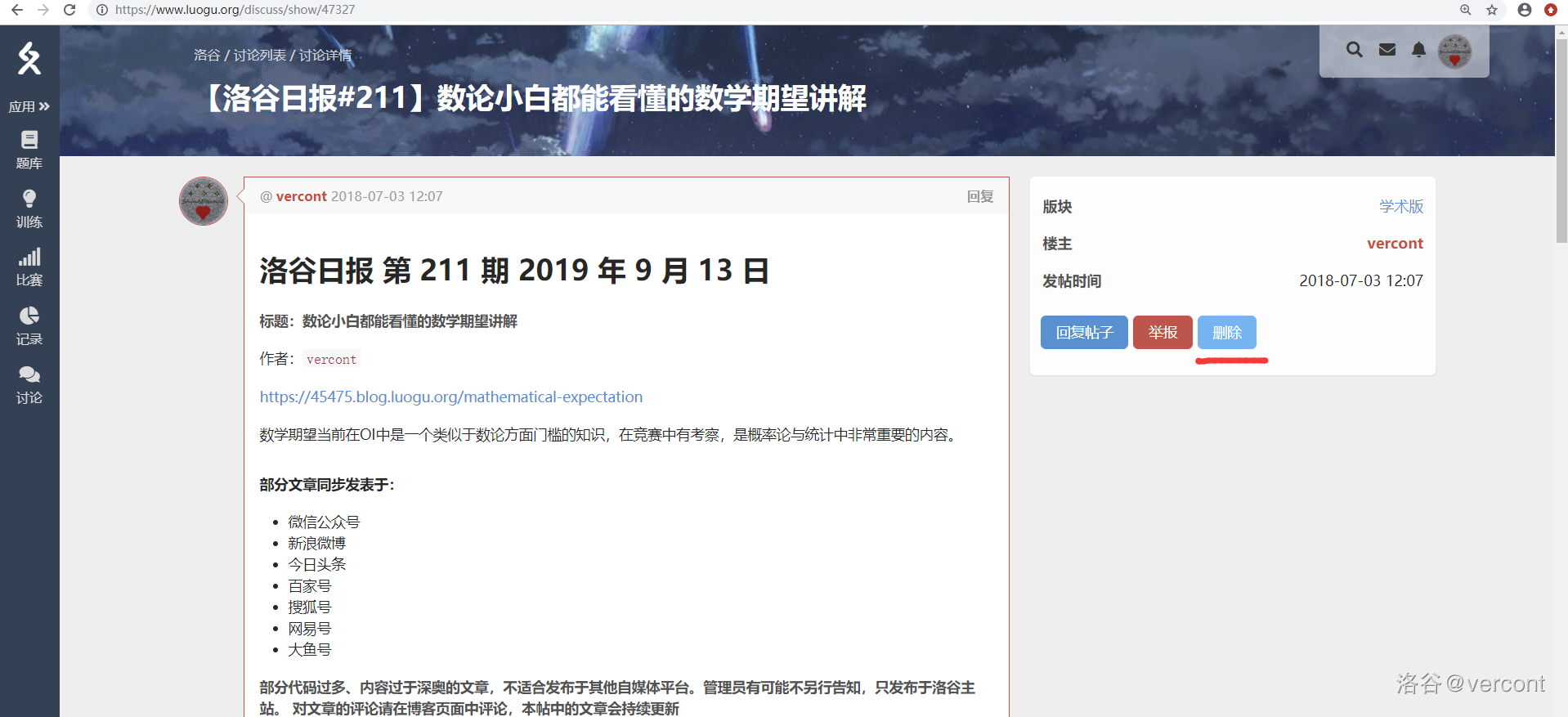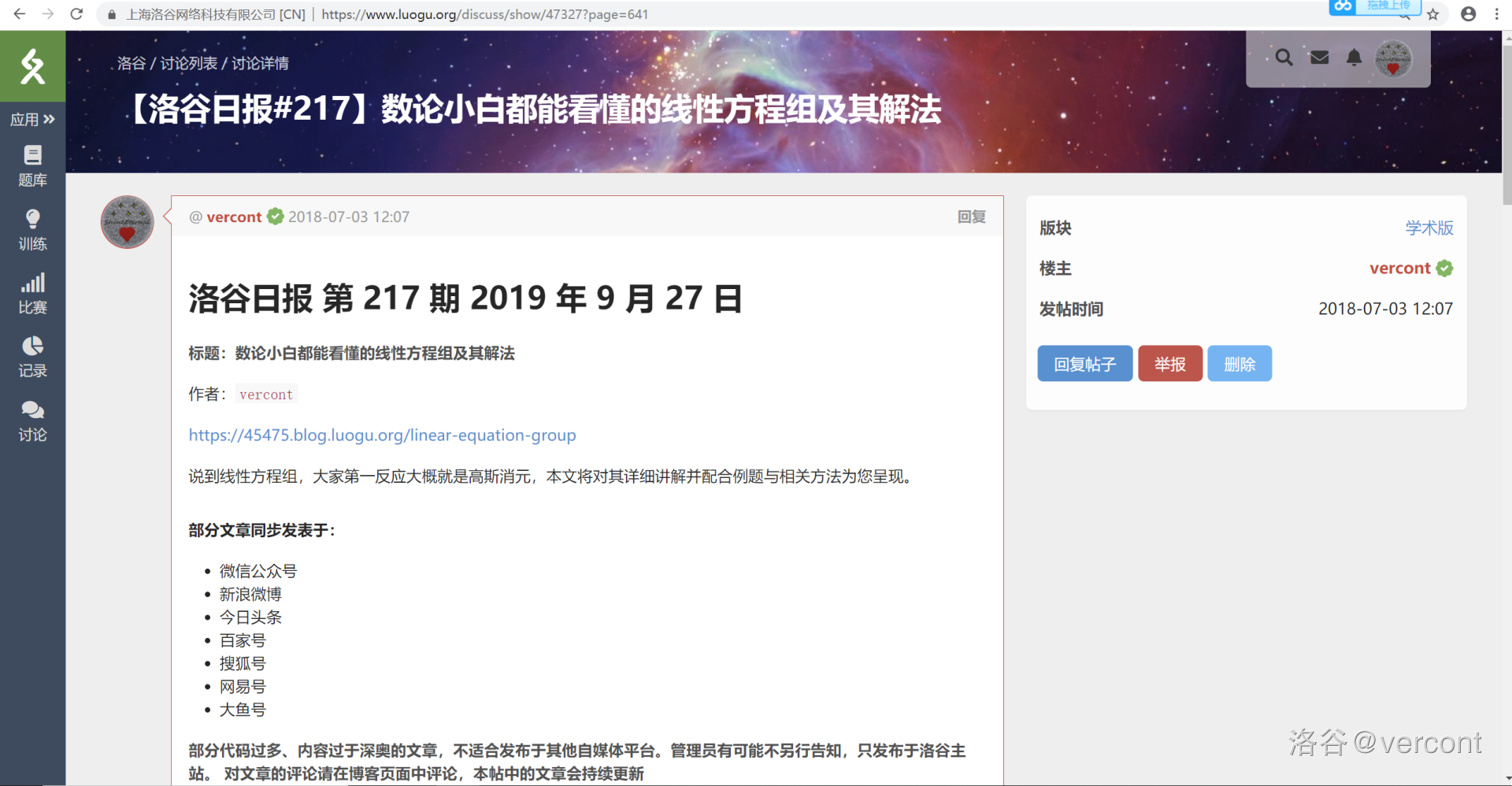# ShineEternal的小书屋# ShineEternal的小书屋

https://blog.csdn.net/kkkksc03

### 洛谷个人主页

posted on 2019-08-30 08:27:42 | under 主页 |# 基本状况：

### 这个用户还有一个常用名 $:^{S^{h^{i^{n^e}}}}_{E_{t_{e_{r_{n_{a_l}}}}}}$

1725• 犇犇：不会参与讨论，只会偶尔祭一些东西

• 灌水区：基本不看

• 学术版和各题目板块会看的，一般也会解答一些问题（雾

• 私信：只要你没被我屏蔽就可以

# 自闭栏

• 还是太菜了QAQ

• 菜是原罪，有bb,感叹人生,互吹互膜以及给自己打气的时间不如现在就清楚的意识到自己是个渣渣的本质

• 然后去刷几道题来提升一下你本来就没多少的水平

• 可怜，弱小，又无助

• 只会裸暴力，还会有些题无从下手

AFO是不可能的，这辈子不可能的，文化课又不会做，只有做点OI这样子才能维持的了生活。

# 成就栏：

50题一更

133

## 9、给洛谷贡献的题目（非比赛贡献）

P5512 棋盘问题（2）【加强】

ps:成就栏统计数据非实时更新

# 大事祭：

• $2019.8.29:$ 通过 $100 {\color{blue} blue}$

• $2019.9.1:$ 期初考试开始&结束，准备原地自爆

• $2019.9.1:$ 开学大凶（相当于报道大凶？）

• $2019.9.2:$ 咕值200整祭

• $2019.9.6:$ 换纯手绘头像祭

• $2019.9.6:$

• $2019.9.9:$

• $2019.9.13:$ 洛咕日报登刊：(存活 $3days$ +)• $2019.9.14:$ 第一次登入洛谷新域名

• $2019.9.21:$

https://www.jisuanke.com/contest/3965?view=rank

13:35左右，经历了半个小时的挣扎，AK了人生第一场网络比赛 $NO.10$ ）

• $2019.9.21:$

• $2019.9.22:$

13:49:审核完毕（4级）

• $2019.9.24:$

• $2019.9.26:$ 打卡400天祭

• $2019.9.27:$• $2019.10.4:$

• $2019.10.6:$